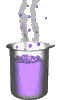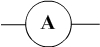Science class online
Chemistry and physics lessons

# Electricity

Free online electricity lessons for elementary school,  middle school and high school.Science class Chemistry Electricity Optics Mechanics Electricity lessons Electrical components -Two-terminal electronic components - Basic electrical components - Diodes - What's a resistor ? - How to determine a resistor value ? - Resistors effects in circuits - How to use a resistor ? - Characteristic curve of a resistor How to build simple circuits and draw diagrams - How to build a basic electrical circuit - How to draw diagrams of electric circuits The electric current - Conductors and insulators - Direction of electric current in a circuit - The dangers of electricity - Current intensity - units how to measure current ? The Voltage - The voltage and its units - How to measure a voltage ? - Voltage in open and closed circuits - Rated current and voltage for a lamp Alternating voltage and current - Alternating currents effects on led - What are alternating current and voltage ? - Periodic alternating voltage and its properties - Oscilloscope - Oscillogram - Frequency - Measuring RMS voltage with a voltmeter Serie circuits - What's a serie circuit ? - Series circuit properties - Short circuit in  series circuit - Current law in series - circuits - Voltage law in series circuits Parallel circuits - What's a parallel circuit ? - Some parallel circuits properties - Short circuits in parallel circuits - Nodes and branches in parallel circuits - Current laws in parallel circuits - Voltage laws in parallel circuits Laws of electricity - Ohm's law - Current laws in series circuits - Voltage laws in series circuits - Current laws in parallel circuits - Voltage laws in parallel circuits Generating electricity - Voltage for coil wires What is an alternator ? - Alternating currents and voltages - Generating electricity in power plants Electric power and energy - Electric power and power rating - Electric power consumption by an electrical device - Relationship between - Electric power and energy

 _____________________________________________________   _____________________________________________________

## The electric current

### How to measure current

 1) The multimeter and ammeter The intensity is measured with an ammeter whose symbol is:The multimeter is a device that can make several kinds of measures including current measurements. We must then use the ammeter mode. 2) How to connect a multimeter in ammeter mode? - Terminals: We use the COM terminal and a terminal current (mA or A) - The sense of connection: The electric current must  always go through the terminal intensity and exit through the COM terminal. It is equivalent to say that the COM terminal is closest to the negative terminal and the terminal current closest to the positive terminal of the electric generator. - The insertion in the circuit: the multimeter is connected in series (The multimeter is inserted between two electric components). ______________________________________ ______________________________________ 3) How to choose the range of the multimeter in ammeter mode? Definition: the chosen range on the ammeter corresponds to the greatest current that it can measure. The selected range should not be smaller than the current because: the measure couldn't be done and the ammeter may be damaged. The range should not be too high otherwise the measurement may lack of accuracy. Method: We must first use the highest range for an approximation of current and then choose the closest (but higher) to obtain a more accurate measurement. ______________________________________ ______________________________________
____________________________________

____________________________________